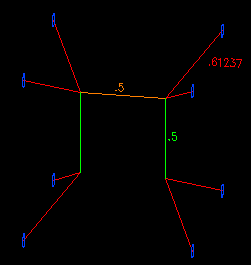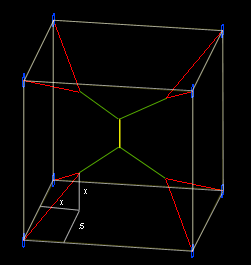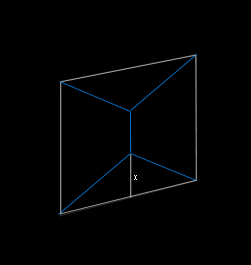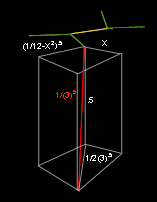BrainDen.com - Brain Teasers

## QuestionA spider is going to weave its new territory attaching to all the tips of wine corks
at the cellar that are forming the 8 corners of a perfect cube (1 cu.m). The spider
must conserve its web fluid (with least linear meter used) and  move from cork to cork.
Can you describe the shape of its web? How long it is?

## Recommended Posts

• 0

It appears the shortest overall length of spinneret thread would be ≈ 6.196152 meters.
The red lines in Plainglazed figure would each be √3/3 meters in length, the green lines would each be √3/6 meters in length, and the central yellow line would be (1 - √3/3) meters in length. This should total (3 + 9√3)/3 meters.

##### Share on other sites

• 0

Connecting the 8 corks with web with least linear meter requires connecting with the web along the sides of the cube (no diagonals) with length 1m, and uses at least 7 segments to connect 8 corks. so the total length is 7*1m = 7 meters. and the shape could vary, as long as you pass the web only along the sides of the cube.

•1
##### Share on other sites

• 0

Welcome here Philip..

Note that the spider can crawl to any cork without spending its web. It would cut and stick web ends anywhere .

Like the previous problem 7m is the length to beat.

What is the minimum length that should connect 3 points?

##### Share on other sites

• 0

just guesing without doing calculation.

Spoiler•1
##### Share on other sites

• 0

Spoiler for one calculation

using nine segments of equal length yields 3(10.5 - 1) or ~6.4868

That is if my math holds up.

##### Share on other sites

• 0

Using the strategy I used in the first connect the dots, this is my answer.

Spoiler

Connect 4 corks with a spread out X. All 4 sides of the X are spread outward to make a 0.5 meter line connecting the two sides. Change the diagonal sides so that they also go up 0.25 meters. Do the same for the top half and connect them with a 0.5 meter line.

The diagonal sides are equal to sqrt(0.375). Multiply that by 8 for all 8 of those lines, and add the connecting 1.5 meters. This is equal to about 6.399 meters.

##### Share on other sites

• 0
Spoiler

Yes, the idea of uniformity and ideal no. of segments are  criteria for its webwork.
@Bb3k
Web has weight ..so the horizontal member shall be connecting web ends of upper diagonals.But the web should be wind resistant somehow. Can you see where the slacks here?

##### Share on other sites

• 0

adding Bb3k's headless stick figure and crunching some numbers:8(2x2+.52).5+ 4(1-2x)/3.5+ 1-2x-(1-2x)/3.5

f(x) = 8(2x2+1/4).5 + 3(1-2x)/3.5 + 1–2x

f’(x) = 16x/(2x2+1/4).5 – 2(3.5) – 2

f’(x) = 0 when x = ~.195

f(x=.195) = 4.568 + 1.409 + .258 = 6.235

the sqrt(3) comes from TSLF's 2D connect the dots:f(x) = 4(x2+.52).5 + 1 – 2x

f”(x) = 4x/(x2+1/4).5 – 2

f’(x) = 0 when x = 1/2(3.5) = ~.289

tan-1(1/2(3.5)/.5) or tan-1(1/3.5) = 30°

##### Share on other sites

• 0

@jasen

Spoiler

You described the shape correctly! (see previous post)..All you need to do is shorten plainglazed figure.

##### Share on other sites

• 0

Spoiler for some supporting math

I have the same calculations as DejMar but with an assumption that I cannot yet prove yields a minimum web length.  In my last response I thought this problem must have some grand symmetry and assumed the red lines in my drawing (above) must be the same distance x from their nearest two sides of the cube described by the eight corks. The following assumes a magical 30 degree angle of those red lines:f(x) = 8/sqrt(3) + 4(1-2x)/sqrt(3) + 1-2sqrt(1/12-x2)-(1-2x)/sqrt(3)
f(x) = 8/sqrt(3) + 3(1-2x)/sqrt(3) + 1-2sqrt(1/12-x2)
f(x) = 8/sqrt(3) + sqrt(3)(1-2x) + 1-2sqrt(1/12-x2)
f'(x) = -2sqrt(3) + 2x/sqrt(1/12-x2)
f'(x) = 0 when x = .25

f(x=.25) = 1+9/sqrt(3) ≈ 6.196

##### Share on other sites

• 0

Alright, you got it.. I don't know if the spider can do that . Maybe with its  farthest pair of eyes.
All webs made a 120 degrees angle with the adjacent web like the towns & roads problem.

You can always minimize piping or wiring lengths using the same principle. The only task is
how to find the points to make 120deg network to connect N dots.

Again, good solving to all .. thanks.

## Join the conversation

You can post now and register later. If you have an account, sign in now to post with your account.×   Pasted as rich text.   Paste as plain text instead

Only 75 emoji are allowed.

×   Your previous content has been restored.   Clear editor

×   You cannot paste images directly. Upload or insert images from URL.